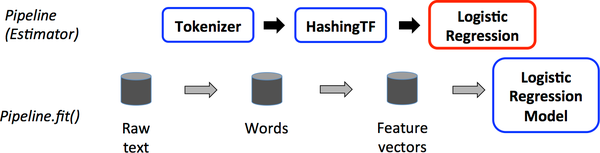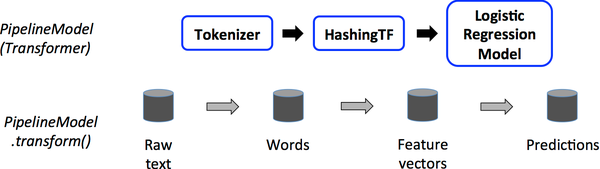## spark ML ，1概述：评估器，转换器和管道 转

spark.ml包目标是提供统一的高级别的API，这些高级API建立在DataFrame上，DataFrame帮助用户创建和调整实用的机器学习管道。在下面spark.ml子包指导中查看的算法指导部分，包含管道API独有的特征转换器，集合等。

Main concepts in Pipelines （管道中的主要概念）

• DataFrame：spark ML从spark sql中获取DataFrame作为学习的数据集。可以持有多种数据类型 ，例如：有个df可以包含文本，特征向量，true标签和预测结果的多个不同的列。

• 转换器： 转换器是把一个DF转成另外一个DF的算法，例：一个ML模型是一个转换器 ，转换带有特征的DataFrame成为带有预测结果的DF.

• 评估器： 评估器是应用（fit）在一个DF上生成一个转换器的算法。 例：学习算法是一个评估器，在DF上训练并且生成一个模型。

• 管道： 一个管道连接多个转换器和评估器在一起，作为一个工作流。

• 参数： 对于指定的参数来说，所有的转换器和评估器 共享一个公共的api.

DataFrame

df支持很多基本和结构化的类型；支持spark sql里的类型，还有向量。
DF可以显性或隐性的从常规的RDD创建。 详见代码
df中的列已经命名了。下面的实例代码中使用的名称 如 “text,” “features,” 和“label.”

• 一个特征转换器拿到一个DF,读取一列映射到一个新列上。 然后输出一个包含映射列的新的DF.
• 一个学习模型拿到一个DF.读取包含特征向量的列，为每个特征向量预测标签， 然后把预测标签作为一个新列放到DF中输出。

Transformer.transform()s and Estimator.fit()s 都是无状态的， 未来，有状态算法可以通过替代概念来支持。

• 把文档中的文本分成单词。
• 转换文档中的每个单词成为数字化的特征向量
• 使用特征向量和标签学习预测模型。

spark ML 描述一个工作流作为一个管道。管道由一系列的PipelineStage(Transformers 和Estimators)组成，以指定的顺序运行。在这部分中我们将使用这个简单的工作流作为运行的例子

How it worksPipeline 和 PipelineModel 有助于确保训练集和测试集得到相同的特征处理步骤。

DAG管道： 一个管道里的多个stage被指定为一个有序的数组，上面的例子给的是线性管道的例子，管道里的stage使用的数据是它上一个stage产生的。也可以创建非线性的管道，只要数据流图是一个DAG图。DAG图可以基于每个stage 输入和输出的列名隐式的指定。如果管道是DAG的形式，然后stage的拓扑图顺序必须被指定。

ML的 Estimator和Transformer 使用相同的api来指定参数。

1.为实例设置参数，例：如果lr是LogisticRegression的实例。设置 lr.setMaxIter(10)，可以让 lr.fit()最多十次迭代。这个API整合了spark.mllib包里的api。
2.传递ParamMap给fit() or transform()方法， ParamMap里的参数将覆盖前面通过setter方法设定的参数。

## Example: Estimator, Transformer, and Param

``````import org.apache.spark.ml.classification.LogisticRegression
import org.apache.spark.ml.param.ParamMap
import org.apache.spark.mllib.linalg.{Vector, Vectors}
import org.apache.spark.sql.Row
``````
``````// Prepare training data from a list of (label, features) tuples.
//准备带标签和特征的数据
val training = sqlContext.createDataFrame(Seq(
(1.0, Vectors.dense(0.0, 1.1, 0.1)),
(0.0, Vectors.dense(2.0, 1.0, -1.0)),
(0.0, Vectors.dense(2.0, 1.3, 1.0)),
(1.0, Vectors.dense(0.0, 1.2, -0.5))
)).toDF("label", "features")

// Create a LogisticRegression instance.  This instance is an Estimator.
//创建一个逻辑回归事例，这个实例是评估器
val lr = new LogisticRegression()
// Print out the parameters, documentation, and any default values.
//输出参数等默认值
println("LogisticRegression parameters:\n" + lr.explainParams() + "\n")

// We may set parameters using setter methods.
//使用setter方法设置参数
lr.setMaxIter(10)
.setRegParam(0.01)

// Learn a LogisticRegression model.  This uses the parameters stored in lr.
//使用存储在lr中的参数来，学习一个模型，
val model1 = lr.fit(training)
// Since model1 is a Model (i.e., a Transformer produced by an Estimator),
// we can view the parameters it used during fit().
// This prints the parameter (name: value) pairs, where names are unique IDs for this
// LogisticRegression instance.
//由于model1是一个模型，（也就是，一个评估器产生一个转换器），
// 我们可以看lr在fit()上使用的参数。
//输出这些参数对，参数里的names是逻辑回归实例的唯一id
println("Model 1 was fit using parameters: " + model1.parent.extractParamMap)

// We may alternatively specify parameters using a ParamMap,
// which supports several methods for specifying parameters.
//我们可以使用paramMap选择指定的参数，并且提供了很多方法来设置参数
val paramMap = ParamMap(lr.maxIter -> 20)
.put(lr.maxIter, 30) // Specify 1 Param.  This overwrites the original maxIter. 指定一个参数。
.put(lr.regParam -> 0.1, lr.threshold -> 0.55) // Specify multiple Params. 指定多个参数

// One can also combine ParamMaps.
val paramMap2 = ParamMap(lr.probabilityCol -> "myProbability") // Change output column name 改变输出列的名称
val paramMapCombined = paramMap ++ paramMap2

// Now learn a new model using the paramMapCombined parameters.
// paramMapCombined overrides all parameters set earlier via lr.set* methods.
//使用新的参数学习模型。
val model2 = lr.fit(training, paramMapCombined)
println("Model 2 was fit using parameters: " + model2.parent.extractParamMap)

// Prepare test data.
//准备测试数据
val test = sqlContext.createDataFrame(Seq(
(1.0, Vectors.dense(-1.0, 1.5, 1.3)),
(0.0, Vectors.dense(3.0, 2.0, -0.1)),
(1.0, Vectors.dense(0.0, 2.2, -1.5))
)).toDF("label", "features")

// Make predictions on test data using the Transformer.transform() method.
// LogisticRegression.transform will only use the 'features' column.
// Note that model2.transform() outputs a 'myProbability' column instead of the usual
// 'probability' column since we renamed the lr.probabilityCol parameter previously.
//使用转换器的transform()方法在测试数据上作出预测.
// 逻辑回归的transform方法只使用“特征”列.
// 注意model2.transform()方法输出的是myProbability列而不是probability列，因为在上面重命名了lr.probabilityCol 参数。
model2.transform(test)
.select("features", "label", "myProbability", "prediction")
.collect()
.foreach { case Row(features: Vector, label: Double, prob: Vector, prediction: Double) =>
println(s"(\$features, \$label) -> prob=\$prob, prediction=\$prediction")
}
``````

## Example: Pipeline

``````import org.apache.spark.ml.{Pipeline, PipelineModel}
import org.apache.spark.ml.classification.LogisticRegression
import org.apache.spark.ml.feature.{HashingTF, Tokenizer}
import org.apache.spark.mllib.linalg.Vector
import org.apache.spark.sql.Row
``````
``````// Prepare training documents from a list of (id, text, label) tuples.
//准备训练文档，（id，内容，标签）
val training = sqlContext.createDataFrame(Seq(
(0L, "a b c d e spark", 1.0),
(1L, "b d", 0.0),
(2L, "spark f g h", 1.0),
)).toDF("id", "text", "label")

// Configure an ML pipeline, which consists of three stages: tokenizer, hashingTF, and lr.
//配置ML管道，由三个stage组成，tokenizer, hashingTF, and lr ，
val tokenizer = new Tokenizer()
.setInputCol("text")
.setOutputCol("words")
val hashingTF = new HashingTF()
.setNumFeatures(1000)
.setInputCol(tokenizer.getOutputCol)
.setOutputCol("features")
val lr = new LogisticRegression()
.setMaxIter(10)
.setRegParam(0.01)
val pipeline = new Pipeline()
.setStages(Array(tokenizer, hashingTF, lr))

// Fit the pipeline to training documents.
//安装管道到数据上
val model = pipeline.fit(training)

// now we can optionally save the fitted pipeline to disk
//现在可以保存安装好的管道到磁盘上
model.save("/tmp/spark-logistic-regression-model")

// we can also save this unfit pipeline to disk
//也可以保存未安装的管道到磁盘上
pipeline.save("/tmp/unfit-lr-model")

// and load it back in during production
//加载管道

// Prepare test documents, which are unlabeled (id, text) tuples.
//准备测试文档，不包含标签
val test = sqlContext.createDataFrame(Seq(
(4L, "spark i j k"),
(5L, "l m n"),
(6L, "mapreduce spark"),
)).toDF("id", "text")

// Make predictions on test documents.
//在测试文档上做出预测
model.transform(test)
.select("id", "text", "probability", "prediction")
.collect()
.foreach { case Row(id: Long, text: String, prob: Vector, prediction: Double) =>
println(s"(\$id, \$text) --> prob=\$prob, prediction=\$prediction")
}
``````

## Example: model selection via cross-validation 通过交叉验证选择模型

RegressionEvaluator评估器Evaluator来评估回归问题，BinaryClassificationEvaluator 来评估二元数据，MultiClassClassificationEvaluator 评估多元分类问题。

``````import org.apache.spark.ml.Pipeline
import org.apache.spark.ml.classification.LogisticRegression
import org.apache.spark.ml.evaluation.BinaryClassificationEvaluator
import org.apache.spark.ml.feature.{HashingTF, Tokenizer}
import org.apache.spark.ml.tuning.{ParamGridBuilder, CrossValidator}
import org.apache.spark.mllib.linalg.Vector
import org.apache.spark.sql.Row
``````
``````    // Prepare training data from a list of (id, text, label) tuples.
//准备训练数据，id 内容，标签
val training = sqlContext.createDataFrame(Seq(
(0L, "a b c d e spark", 1.0),
(1L, "b d", 0.0),
(2L, "spark f g h", 1.0),
(4L, "b spark who", 1.0),
(5L, "g d a y", 0.0),
(6L, "spark fly", 1.0),
(7L, "was mapreduce", 0.0),
(8L, "e spark program", 1.0),
(9L, "a e c l", 0.0),
(10L, "spark compile", 1.0),
)).toDF("id", "text", "label")

// Configure an ML pipeline, which consists of three stages: tokenizer, hashingTF, and lr.
//    配置机器学习管道，由tokenizer, hashingTF,  lr评估器 组成
val tokenizer = new Tokenizer()
.setInputCol("text")
.setOutputCol("words")
val hashingTF = new HashingTF()
.setInputCol(tokenizer.getOutputCol)
.setOutputCol("features")
val lr = new LogisticRegression()
.setMaxIter(10)
val pipeline = new Pipeline()
.setStages(Array(tokenizer, hashingTF, lr))

// We use a ParamGridBuilder to construct a grid of parameters to search over.
// With 3 values for hashingTF.numFeatures and 2 values for lr.regParam,
// this grid will have 3 x 2 = 6 parameter settings for CrossValidator to choose from.
//使用ParamGridBuilder 构造一个参数网格，
//hashingTF.numFeatures有3个值，lr.regParam有2个值，
// 这个网格有6个参数给CrossValidator来选择
val paramGrid = new ParamGridBuilder()
.build()

// We now treat the Pipeline as an Estimator, wrapping it in a CrossValidator instance.
// This will allow us to jointly choose parameters for all Pipeline stages.
// A CrossValidator requires an Estimator, a set of Estimator ParamMaps, and an Evaluator.
// Note that the evaluator here is a BinaryClassificationEvaluator and its default metric
// is areaUnderROC.
//现在我们把管道看做成一个Estimator，把它包装到CrossValidator实例中。
//这可以让我们连带的为管道的所有stage选择参数。
//CrossValidator需要一个Estimator，一个评估器参数集合，和一个Evaluator。
//注意这里的evaluator 是二元分类的BinaryClassificationEvaluator，它默认的度量是areaUnderROC.
val cv = new CrossValidator()
.setEstimator(pipeline)
.setEvaluator(new BinaryClassificationEvaluator)
.setEstimatorParamMaps(paramGrid)
.setNumFolds(2) // Use 3+ in practice  // 在实战中使用3+

// Run cross-validation, and choose the best set of parameters.
//运行交叉校验，选择最好的参数集
val cvModel = cv.fit(training)

// Prepare test documents, which are unlabeled (id, text) tuples.
//准备测试数据
val test = sqlContext.createDataFrame(Seq(
(4L, "spark i j k"),
(5L, "l m n"),
(6L, "mapreduce spark"),
)).toDF("id", "text")

// Make predictions on test documents. cvModel uses the best model found (lrModel).
//在测试文档上做预测，cvModel是选择出来的最好的模型
cvModel.transform(test)
.select("id", "text", "probability", "prediction")
.collect()
.foreach { case Row(id: Long, text: String, prob: Vector, prediction: Double) =>
println(s"(\$id, \$text) --> prob=\$prob, prediction=\$prediction")
}
``````

## Example: model selection via train validation split 例：通过训练校验分离来模型选择

TrainValidationSplit 需要传入一个Estimator,一个包含estimatorParamMaps 参数的paraMap的集和一个Evaluator。它一开始使用trainRatio 参数值把数据集分成训练数据和测试数据两个部分。例如： 使用trainRatio=0.75 （默认值），TrainValidationSplit 就产生75%数据用于训练，25%的数据用于测试。与CrossValidator相似的是，TrainValidationSplit 也是通过迭代参数集paramMap。对于每一种参数组合，使用给定的Estimator 训练，在给定 Evaluator上评估。产生最好的评估度量的paramMap作为最好的选择。TrainValidationSplit 最终会使用最好的参数和整个数据集条用Estimator的fit方法。

``````import org.apache.spark.ml.evaluation.RegressionEvaluator
import org.apache.spark.ml.regression.LinearRegression
import org.apache.spark.ml.tuning.{ParamGridBuilder, TrainValidationSplit}
``````
``````// Prepare training and test data.
//准备训练数据和测试数据
val Array(training, test) = data.randomSplit(Array(0.9, 0.1), seed = 12345)

val lr = new LinearRegression()

// We use a ParamGridBuilder to construct a grid of parameters to search over.
// TrainValidationSplit will try all combinations of values and determine best model using
// the evaluator.
//ParamGridBuilder构建一组参数
//TrainValidationSplit将尝试从这些所有值的组合中使用evaluator选出最好的模型
val paramGrid = new ParamGridBuilder()
.build()

// In this case the estimator is simply the linear regression.
// A TrainValidationSplit requires an Estimator, a set of Estimator ParamMaps, and an Evaluator.
//在这里estimator是简单的线性回归
//TrainValidationSplit 需要一个Estimator ， 一个Estimator ParamMaps集，一个Evaluator
val trainValidationSplit = new TrainValidationSplit()
.setEstimator(lr)
.setEvaluator(new RegressionEvaluator)
.setEstimatorParamMaps(paramGrid)
// 80% of the data will be used for training and the remaining 20% for validation.
//80%数据作为训练，剩下的20%作为验证
.setTrainRatio(0.8)

// Run train validation split, and choose the best set of parameters.
//运行训练校验分离，选择最好的参数。
val model = trainValidationSplit.fit(training)

// Make predictions on test data. model is the model with combination of parameters
// that performed best.
//在测试数据上做预测，模型是参数组合中执行最好的一个
model.transform(test)
.select("features", "label", "prediction")
.show()
``````

### 评论(0)

1 MLlib概述 1.1 MLlib 介绍 ◆ 是基于Spark core的机器学习库,具有Spark的优点 ◆ 底层计算经过优化,比常规编码效率往往要高 ◆ 实现了多种机器学习算法,可以进行模型训练及预测 1.2 Spark ...

javaedge
2019/04/09
0
0
Spark的Ml pipeline理解

ML pipeline提供了一组统一的高级API，它们构建在 DataFrame之上，可帮助用户创建和调整实用的机器学习pipeline。 一 重要概念 1.1 管道中的主要概念 MLlib对机器学习算法的API进行了标准化，...

lirika_777
03/31
0
0
spark 与 scikit-learn 机器学习流程组件设计哲学比较

osc_9z0e3l8u
2018/11/18
2
0

SparkNLP的官方文档 1>sbt引入: 2>maven引入：　 < dependency >< groupId > com.johnsnowlabs.nlp </ groupId >< artifactId > spark-nlp_2.11 </ artifactId >< version > 1.4.2 </ versi......

osc_v2gt986j
2018/03/19
2
0
Spark的MLlib和ML库的区别

osc_zrrg1637
2018/02/27
8
0

IntelliJ 安装 Python 插件

honeymoose
3分钟前
4
0

osc_5p8bxoq2
3分钟前
6
0
ASP.NET Core MVC如何上传文件及处理大文件上传

osc_yq7pxbpa
4分钟前
8
0
ASP.NET Core MVC如何上传文件及处理大文件上传

osc_dihgscoq
6分钟前
9
0

该炸的 Starship 原型机总是会炸的，该进行的载人任务也总是会发射的。 　　承载着 SpaceX 未来星际旅行梦想的 Starship 宇宙飞船原型机再一次在测试中以全部炸毁收场。 　　 　　但需要...

osc_35ne77sz
7分钟前
7
0